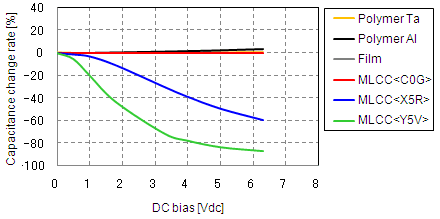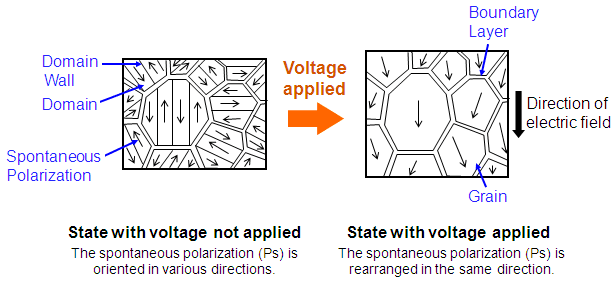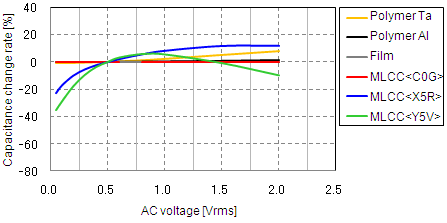Capacitor Guide

# The voltage characteristics of electrostatic capacitance

Greetings, everyone.
This technical column describes the basic facts about capacitors.
This lesson describes the voltage characteristics of electrostatic capacitance.

## Voltage characteristics

The phenomenon where the effective capacitance value of a capacitor changes according to the direct current (DC) or alternating current (AC) voltage is called the voltage characteristics. Capacitors are said to have good voltage characteristics when this variance width is small, or poor temperature characteristics when the variance width is large. When using capacitors in electronic equipment used for applications such as ripple rejection in power lines, the design must take into account the operating voltage conditions.

### 1. DC bias characteristic

DC bias characteristic refers to the phenomenon where the effective electrostatic capacitance changes (decreases) when DC voltage is applied to a capacitor. This phenomenon is peculiar to high dielectric constant-type multilayer ceramic capacitors that use barium titanate-based ferroelectrics, and does not occur much at all in conductive polymer aluminum electrolytic capacitors (Polymer Al), conductive polymer tantalum electrolytic capacitors (Polymer Ta), film capacitors (Film), and temperature-compensating-type multilayer ceramic capacitors that use titanium oxide or calcium zirconate-based paraelectrics (MLCC<C0G>) (see Figure 1).
I will use an example to explain what actually occurs. Imagine the case where DC voltage of 1.8 V is applied to a high dielectric constant-type multilayer ceramic capacitor with a rated voltage of 6.3 V and an electrostatic capacitance of 100 uF. In this case, the electrostatic capacitance of a product with X5R temperature characteristics decreases by approximately 10%, so the effective capacitance value becomes 90 uF. In addition, the electrostatic capacitance of a Y5V characteristics product decreases by approximately 40%, so the effective capacitance value becomes 60 uF.Figure 1. Capacitance change rate vs. DC bias characteristics of various capacitor types (Example)

When DC voltage is applied to a barium titanate-based ferroelectric, the electric flux density (D) and the electric field (E) are proportional when the electric field is small. However, as the electric field increases, the spontaneous polarization (Ps) that was oriented in various directions begins to rearrange in the direction of the electric field, the material exhibits an extremely large dielectric constant, and the effective capacitance value increases. When the electric field increases further to the point where spontaneous polarization rearrangement ends and the polarization becomes saturated, the dielectric constant becomes smaller and the effective capacitance value decreases (see Figure 2).
For this reason, when selecting multilayer ceramic capacitors, the electrostatic capacitance noted in the catalog should not be accepted without question. Instead, it is necessary to measure the electrostatic capacitance while applying the DC voltage component of the power supply (signal) line where the capacitor is to be used, and understand the effective capacitance value. However, this DC bias characteristic is such that the amount of decrease in the electrostatic capacitance becomes smaller as the applied DC voltage component decreases. Recently, semiconductor chips such as FPGA and ASIC that operate with a supply voltage (DC voltage) of less than 1 V have been appearing, and issues related to DC bias characteristics are not so noticeable for multilayer ceramic capacitors used in the power supply lines of these semiconductor chips.Figure 2. State when voltage is applied to a ferroelectric ceramic

### 2. AC voltage characteristic

AC voltage characteristic refers to the phenomenon where the effective electrostatic capacitance changes (increases or decreases) when AC voltage is applied to a capacitor. Like the DC bias characteristic, this phenomenon is peculiar to high dielectric constant-type multilayer ceramic capacitors that use barium titanate-based ferroelectrics, and does not occur much at all in conductive polymer aluminum electrolytic capacitors (Polymer Al), conductive polymer tantalum electrolytic capacitors (Polymer Ta), film capacitors (Film), and temperature-compensating-type multilayer ceramic capacitors that use titanium oxide or calcium zirconate-based paraelectrics (MLCC<C0G>) (see Figure 3).
For example, imagine the case where AC voltage (Frequency: 120 Hz) of 0.2 Vrms is applied to a high dielectric constant-type multilayer ceramic capacitor with a rated voltage of 6.3 V and an electrostatic capacitance of 22 uF. In this case, the electrostatic capacitance of a product with X5R temperature characteristics decreases by approximately 10%, so the effective capacitance value becomes 20 uF. In addition, the electrostatic capacitance of a Y5V characteristics product decreases by approximately 20%, so the effective capacitance value becomes 18 uF.Figure 3. Capacitance change rate vs. AC voltage characteristics of various capacitor types (Example)

As described above, the grains of ferroelectric ceramics have domains, and the spontaneous polarization (Ps) of each domain is oriented randomly, which is equivalent to the state without overall polarization. When an electric field (E) is applied to a ferroelectric ceramic in this state, the polarization aligns in the direction of the electric field and reaches the saturation value. Even when the electric field is removed from this state, the direction of polarization does not return to the original random state, but remains partially in the polarized state, and this appears externally as residual polarization. To return this residual polarization to 0 (zero) requires an electric field with the opposite direction. In addition, when the reverse electric field is strengthened further, polarization reversal occurs and the material is polarized in the opposite direction. In this manner, the polarization behavior of ferroelectrics due to an external electric field draws a D-E hysteresis curve such as shown in Figure 4.
Large waveform distortion occurs in the current flowing to a ferroelectric capacitor under high AC voltage conditions, so the definition of linear materials (*1) cannot be applied as is. However, it can be safely said that the dielectric constant (εr) obtained from the effective capacitance value is roughly equivalent to the average slope of the hysteresis curve (the straight lines indicated by dashed lines in Figure 4).